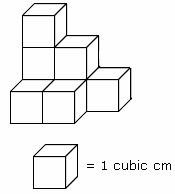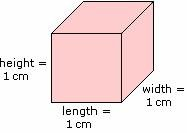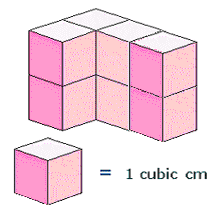## Definition Of Cubic Centimeter

Cubic Centimeter is a standard metric unit used to measure the volume.

Cubic centimeter is denoted as cm3 or cu cmM
1 liter = 1,000 cubic cm.

### Example of Cubic CentimeterThere are 8 cubes in the above figure and the volume of each cube is 1 cubic cm. So, the volume of the figure is 8 cubic cm, as Volume of the shape = number of units × volume of each unit.The volume of the cube V = length × width × height
= 1 cm × 1 cm × 1 cm
= 1 cubic cm

### Solved Example on Cubic Centimeter

#### Ques: Determine the volume of the solid shown in the figure.A. 6 cubic cm
B. 7 cubic cm
C. 8 cubic cm
D. 4 cubic cm